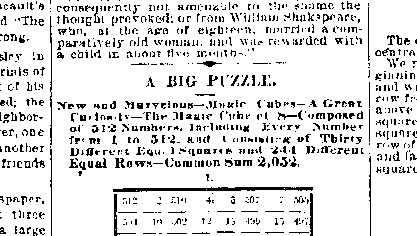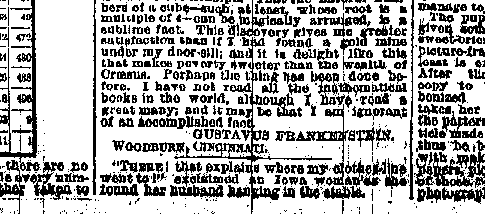Original text of the first published perfect magic cube of order 8, 1875
by Gustavus Frankenstein, "The Cincinnati Commercial" newspaper, March 11th, 1875

A BIG PUZZLE

New and Marvelous - Magic Cubes - A Great Curiosity - The Magic Cube of 8 - Composed of 512 Numbers, Including Every Number from 1 to 512, and Consisting of Thirty Different Equal Squares and 244 Different Equal Rows - Common Sum 2,052512 2 510 4 5 507 7 505 504 10 502 12 13 499 15 497 17 495 19 493 492 22 490 24 25 487 27 485 484 30 482 32 33 479 35 477 476 38 474 40 41 471 43 469 468 46 466 48 464 50 462 52 53 459 55 457 456 58 454 60 61 451 63 449

(Look at the Excel file for the Squares 2 to 8
and for the following Squares A to D)

Throughout these eight squares there are no two numbers alike, but they include every number from 1 to 512. Each row, whether taken to the right and left, up and down, or from corner to opposite corner of any one of these squares, foots up the same, being equal to 2,052.

Now place the second square upon the first, the third upon the second, the fourth upon the third, the fifth upon the fourth, the sixth upon the fifth, the seventh upon the sixth, the eighth upon the seventh. We shall then have a cube; and from every one of the sixty-four cells occupied by the numbers of the first square will arise a vertical column, consisting of eight numbers; thus making sixty-four different vertical columns, which will also foot up 2,052. These vertical columns make eight different vertical squares taken from right to left, and eight different vertical squares taken up and down, besides three pairs of different diagonal squares. All these squares are equal, and any row in any one of them will foot up 2,052. To show this, let us make up a new square by taking one transverse row from each of the eight squares. The new square thus formed will differ by the up-and-down or longitudinal rows from any rows found in the original squares; but at the same time each will foot up 2,052. Take for example the third row from the top out of each of the eight original squares, beginning in the square 1 and ending with the square 8:

(Square A)

A new square can be formed from any one of the other seven transverse rows, and eight squares also from the longitudinal or up-and-down rows.

A single number can anywhere be taken from the first square, another number from the cell occupying the same position in the second square, a third from the same position in the third, and so on all the way to the eighth. This will form a single row, which will foot up to 2,052. There can thus be formed sixty-four different equal rows. For instance, take in the first square 469, which is in the third row from the bottom and the fourth from the left side; in the second square take 108, occupying the same position; 172 from the third; 277 from the fourth; 213 from the fifth; 364 from the sixth; 428 from the seventh; and 21 from the eighth. These numbers add up 2,052.

Now let us form one of the diagonal squares of the cube. Take the top row of the first square. Write under it the second row of the second square; under that the third row of the third square; under that the fourth row of the fourth square; then the fifth row of the fifth square; the sixth row of the sixth, the seventh row of the seventh, and lastly the eighth or bottom row of the eighth square:

(Square B)

The diagonals of B are one pair of the four central diagonals of the cube.

We may write another diagonal square by beginning with the bottom row of the first square and writing above it, or under it, the second row from the bottom of the second square; above that again the third row of the third square; then the fourth row of the fourth square; then the fifth row of the fifth, the sixth row of the sixth, the seventh row of the seventh, and lastly the eighth or top row of the eighth square. Thus:

(Square C)

The square B contains one pair of the four central diagonals of the cube, and the square C the other pair.

Another diagonal square can be formed by taking the first up and down row on the left of square 1 and ending with the up and down row on the right of square 8; and its companion diagonal square will be formed by taking the right hand up and down row of square 1, proceeding to the left through 2, 3, 4, 5, 6, 7, and ending with the last up and down row on the left of square 8.

Now let us form a diagonal square of the cube by taking a diagonal row from each of the original eight squares. Thus, beginning at the upper left hand corner of the first square, write its diagonal row. Place under it the corresponding diagonal of the second square, then that of the third, fourth, fifth, sixth, seventh, eighth:

(Square D)

I have given twelve squares, but there are in all thirty different squares contained in the cube, and anybody can form, by way of curiosity and amusement, the eighteen squares not given in this article. The thirty squares really exist by virtue of the eight original ones, and if the numbers were actually placed in the form of a cube they would be plainly visible, and so also would the 244 distinct rows.

The solution of this wonderful problem is contained in the following little square:

 1 3 4 2 4 2 1 3 2 4 3 1 3 1 2 4

If you can interpret this simple arrangement of four numbers, you will be able to write the eight original squares nearly as fast as figures can be made.

It may be asked, "What was the nature of the calculation employed in solving so intricate a problem?". To this I will answer that neither addition, subtraction, multiplication, or division, nor the employment of a single number or algebraical letter entered into it, unless the four numbers of the scheme be excepted, and the whole cube was magically constructed before a number was written.

I will give you the

 1 5 2 3 6 4 6 2 1 5 4 3 4 6 3 2 1 5 5 3 6 4 2 1 3 1 4 6 5 2 2 4 5 1 3 6

If you could interpret this little square, you could construct the magic cube of 12 (1,728 numbers), after the squares had been briefly prepared, almost as readily and as rapidly as you could write numbers ordinarily.

It was in the pleasant days of the past autumn, during the reign of that delightful weather that makes lovely and beloved Ohio enchantingly beautiful, and to which I must ever pay grateful tribute, that the thought occurred to me: Why can not numbers be arranged in the form of a cube, so that all the rows it may contain shall add up alike? To answer this question was to attempt the solution of the problem. It seemed to me it could be done. The Magic Cube of 8 which I have given, it appears to me, is a sufficient triumph. That the natural numbers of a cube -such, at least, whose root is a multiple of 4- can be magically arranged, is a sublime fact. This discovery gives me greater satisfaction than if I had found a gold mine under my door-sill; and it is delight like this that makes poverty sweeter than the wealth of Craesus. Perhaps the thing has been done before. I have not read all the mathematical books in the world, although I have read a great many; and it may be that I am ignorant of an accomplished fact.

Gustavus Frankenstein
Woodburn, Cincinnati